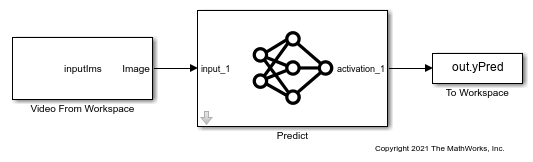# Classify Images in Simulink with Imported TensorFlow Network

This example shows how to import a pretrained TensorFlow™ network in the saved model format by using `importTensorFlowNetwork`, and then use the Predict block to classify a sequence of images in Simulink®. The imported network contains layers that are not supported for conversion into built-in MATLAB® layers. `importTensorFlowNetwork` automatically generates custom layers when you import these layers. The Predict block predicts responses for the data at the input by using the trained network that you specify using the block parameters.

`importTensorFlowNetwork` requires the Deep Learning Toolbox™ Converter for TensorFlow Models support package. If this support package is not installed, then `importTensorFlowNetwork` provides a download link.

Load the digit sample data as an image datastore. `imageDatastore` automatically labels the images based on folder names and stores the data as an `ImageDatastore` object.

```digitDatasetPath = fullfile(matlabroot,"toolbox","nnet","nndemos", ... "nndatasets","DigitDataset"); imds = imageDatastore(digitDatasetPath, ... IncludeSubfolders=true,LabelSource="foldernames");```

For reproducibility, specify the seed for the MATLAB random number generator. Randomly select eight images from the image datastore, create the array of images `inputIms`, and display the selected images by using `montage` (Image Processing Toolbox).

```rng("default") perm = randperm(10000,8); for i = 1:8 inputIms(:,:,:,i) = imread(imds.Files{perm(i)}); end montage(inputIms,size=[1 NaN]);```### Import Pretrained TensorFlow Network

Specify the model folder that contains the pretrained network `digitsDAGnetwithnoise` in the saved model format. `digitsDAGnetwithnoise` can classify images of digits.

```if ~exist("digitsDAGnetwithnoise","dir") unzip("digitsDAGnetwithnoise.zip") end modelFolder = "./digitsDAGnetwithnoise";```

Specify the class names.

`classNames = {'0','1','2','3','4','5','6','7','8','9'};`

Import a TensorFlow network in the saved model format. By default, `importTensorFlowNetwork` imports the network as a `DAGNetwork` object.

`net = importTensorFlowNetwork(modelFolder,Classes=classNames);`
```Importing the saved model... Translating the model, this may take a few minutes... Finished translation. Assembling network... Import finished. ```

Analyze the imported network. `analyzeNetwork` displays an interactive plot of the network architecture and a table containing information about the network layers.

`analyzeNetwork(net)`The imported network contains layers that are not supported for conversion into built-in MATLAB layers. The software automatically generates the custom layers `gaussian_noise_1` and `gaussian_noise_2`. The function `importTensorFlowNetwork` saves each generated custom layer to a separate `.m` file in the package `+digitsDAGnetwithnoise` in the current folder. For more information on these generated custom layers, see Import TensorFlow Network with Autogenerated Custom Layers.

Save the imported network in a MAT file.

```filename = "digitsNet.mat"; save(filename,"net")```

This example provides the Simulink model `slexDigitsImportedNetworkPredictExample.slx`. You can open the Simulink model (provided in this example) or create a new model by following the steps described in this section.

Open the Simulink model `slexDigitsImportedNetworkPredictExample.slx`.

```SimMdlName = "slexDigitsImportedNetworkPredictExample"; open_system(SimMdlName)```1. To create a new Simulink model, open the Blank Model template and add the Predict block from the Deep Learning Toolbox™ library. The Predict block predicts responses for the data at the input by using the trained network that you specify using the block parameters. The input to the block can be an h-by-w-by-c-by-N numeric array, where h, w, and c are the height, width, and number of channels of the images, respectively, and N is the number of images.

Double-click the Predict block to open the Block Parameters dialog box. Select `Network from MAT-file` for the Network parameter. Click Browse in the File Path section to specify the network as the `digitsNet.mat` network in the current folder.2. Insert the Video from Workspace block from the Computer Vision Toolbox™ library. Double-click the Video from Workspace block to open the Block Parameters dialog box. Specify Signal as `inputIms`, Sample time as 1, and Form output after final value by as Holding final value.3. Check if the size of the input images matches the network input size. If they do not match, you must resize the input data by adding the Resize block from the Computer Vision Toolbox library to the model.

Display the size of the image and the input size of the network.

`size(inputIms)`
```ans = 1×4 28 28 1 8 ```
`netInputSize = net.Layers(1).InputSize`
```netInputSize = 1×3 28 28 1 ```

The input is a sequence of eight grayscale (one-channel) images that are 28-by-28 pixels in size. The image size matches the network input size.

4. Add a To Workspace block to the model and change the variable name to `yPred`. Connect the Video from Workspace block to the input of the Predict block and the To Workspace block to the output of the Predict block.

5. Open the Configuration Parameters dialog box. On the Modeling tab, click Model Settings. Under Solver selection, set Type to `Fixed-step`, and set Solver to `discrete (no continuous states)`.

Simulate the model and save the simulation output to `modelOutput`. The field `modelOutput.yPred.Data` contains the classification results.

`modelOutput = sim(SimMdlName)`
```modelOutput = Simulink.SimulationOutput: tout: [8x1 double] yPred: [1x1 timeseries] SimulationMetadata: [1x1 Simulink.SimulationMetadata] ErrorMessage: [0x0 char] ```

Display the sequence of images and the classification results.

```tiledlayout(1,12,TileSpacing="None"); for i = 1:size(inputIms,4) nexttile imshow(inputIms(:,:,:,i)) label = modelOutput.yPred.Data(:,:,i)==1; title([classNames{label}],FontSize=20) end```